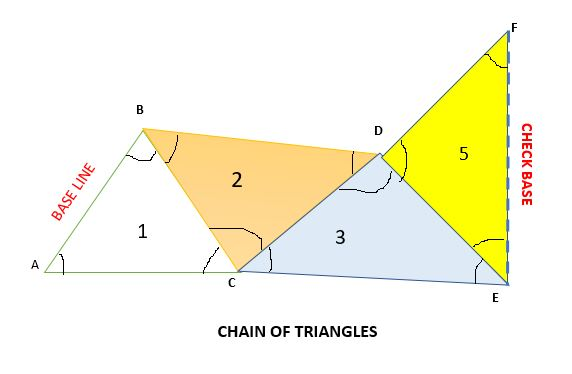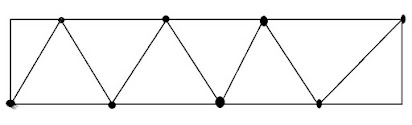# What is Triangulation in Surveying?

Triangulation is a technique followed in surveying to achieve horizontal control. In a triangulation system, a number of interconnected triangles are traced and their angles are measured to determine the relative positions of the points spread over an area.

In this system, the length of only one line is determined, called the baseline and the angles are measured using an instrument, and the length of all the sides is determined using trigonometric formula.## Principal of Triangulation in Surveying

The principle of triangulation in surveying is that "if one side and three angles are known for a triangle, then the remaining sides can be calculated using the sine rule."

In the above figure, if the anyone side for example c, and the angles A, B, and C are known, then as per the sine rule,

a/sin A = b/ sin B = c/ sin C;

No time to Read? 🕮   Watch the Video on What is Triangulation Surveying?

## Triangulation System in Surveying

As mentioned, a series of interconnected triangles form the survey area. If we know the length of one side and the three angles, the length of the other sides of each triangle can be computed.

In this system, the apex of the triangle is called the triangulation station, and the whole figure is called as triangulation system or triangulation figure.

The common types of figures that are used in triangulation systems are:

1. Triangles
3. Polygons
All the figures that are used in the triangulation system for surveying must follow certain conditions. which include:
1. The sum of the interior angles should be (2n-4) x 90, where the number of sides of the figure is given by 'n'.
2. The total sum of the angles joining at a station must be equal to 360 degrees.
3. The length of the sides that are calculated through more than one route should match and agree.

### 1. Triangles for Triangulation

Among all the figures, triangles are most commonly used because they are very rapid and economical when a narrow strip is to be surveyed. The triangles used for triangulation must not have interior angles, less than 30 degrees, and greater than 120 degrees.

This method is more economical, simple, and rapid. But as it is used in a long narrow strip, a number of the baselines is introduced frequently which would result in the accumulation of errors. Hence it is less accurate.#### Triangles for Triangulation

This is an excellent figure system that can involve various combinations of sides and angles. It can be used to compute lengths of required sides and checks can be made frequently.

The best quadrilateral is square. A quadrilateral with both diagonals without a station at their intersection is followed. Quadrilateral figures are used in triangulation for hilly areas. It involves more checks, hence the method is accurate.

### 3. Polygons for Triangulation

For areas that are very wide, their length is surveyed either by a pentagonal or hexagonal area. This option is more economical compared to triangles when the wider area is there.

In the case of polygon figures, they may or may not have a central station. It involves more checks, hence the method is accurate.Polygons with Central Points

## Triangulation Figures or Layouts in Triangulation Survey

A triangulation figure is a group of triangles, with a single and common side to the preceding and following figures used in the triangulation technique in construction surveying.

The common figures or systems used in triangulation systems are:

1. Single Chain of Triangles
2. Double Chain of Triangles
3. Central Point Figures
5. Combination of all the system

### 1. Single Chain of Triangles in Triangulation

A single chain of triangles is used to survey a narrow strip of terrain. This figure or system is rapid and economical. The figures made in this method are not so accurate and are not used for primary works. The number of conditions that are used to fulfill the figure conditions is relatively small.
In this method, two independent routes cannot be used to determine the solution of the triangles. Hence, to reduce the accumulation of errors, it is recommended to introduce a baseline frequently.Single Chain of Triangles

### 2. Double Chain of Triangles

Double-chain figures are used for covering a larger area.

### 3. Centered Figures

It is used to cover the area in a flat country. It provides satisfactory results. The centered figures can be quadrilaterals, hexagons, or pentagons with central stations. The figure provides desired checks during the computations. The progress of centered figures work is slow as they require more instrument setting.

The quadrilateral with four corner stations and observed diagonal forms the best figures. It is best suited for a hilly country. Since the computed length of the sides can be carried through the system by different combinations of sides and angles, the system is the most accurate.

In fact, a braced quadrilateral consists of overlapping triangles. This system is treated to be the strongest and the best arrangement of triangles, and it provides a means of computing the lengths of the sides using different combinations of sides and angles. Most of the triangulation systems use this arrangement

### 5. Combination of all above systems

Sometimes a combination of the above systems may be used which may be according to the shape of the area and the accuracy requirements.

## Where is Triangulation Survey Used?

Triangulation is mainly used for the survey of hills and undulating areas. In these areas, the triangulation method helps to establish stations at reasonable distances apart. The triangulation technique is used in various surveying methods like geodetic surveying, cadastral surveying, topographic surveying, control surveying, and engineering surveying, where the commonly used instruments are theodolites, total stations, GNSS receivers, etc.

But, this method is not suitable for planes and crowded areas, where the intervisibility of stations is affected. In situations, where it is a must, building towers are constructed, but it is costly.

Triangulation is a very precise technique that is used for larger areas, where the angles between the points are relatively small. But for highly accurate requirements, we must go for surveying techniques like aerial mapping, total station surveying, etc.